# Plot¶

## Base¶

### layout¶

For two subplots, the height of the first subplot is 8 times than the height of the second subplot,

layout(mat = matrix(c(rep(1, 8), 2), ncol = 1, byrow = TRUE))


see more details in https://stats.hohoweiya.xyz/2022/11/21/KEGGgraph/

### math formula¶

No need to use paste function ()

COMMAND FIGURE
~ in the expression represents a space: expression(xLab ~ x^2 ~ m^-2)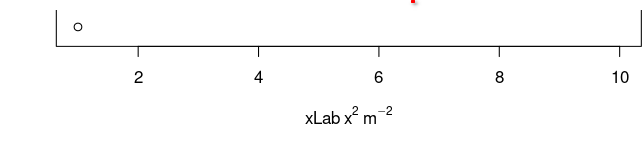* in the expression implies no space: expression(xLab ~ x^2 * m^-2)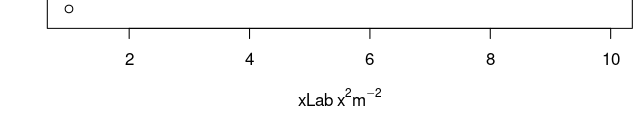expression(R[group("", list(hat(F),F),"")]^2) OR expression(R[hat(F) * ',' ~ F]^2)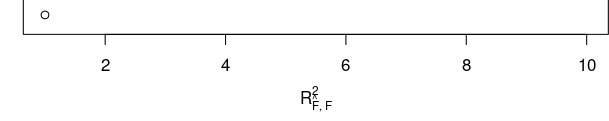### pure figure without axis¶

Suppose I want to draw the following figure with R,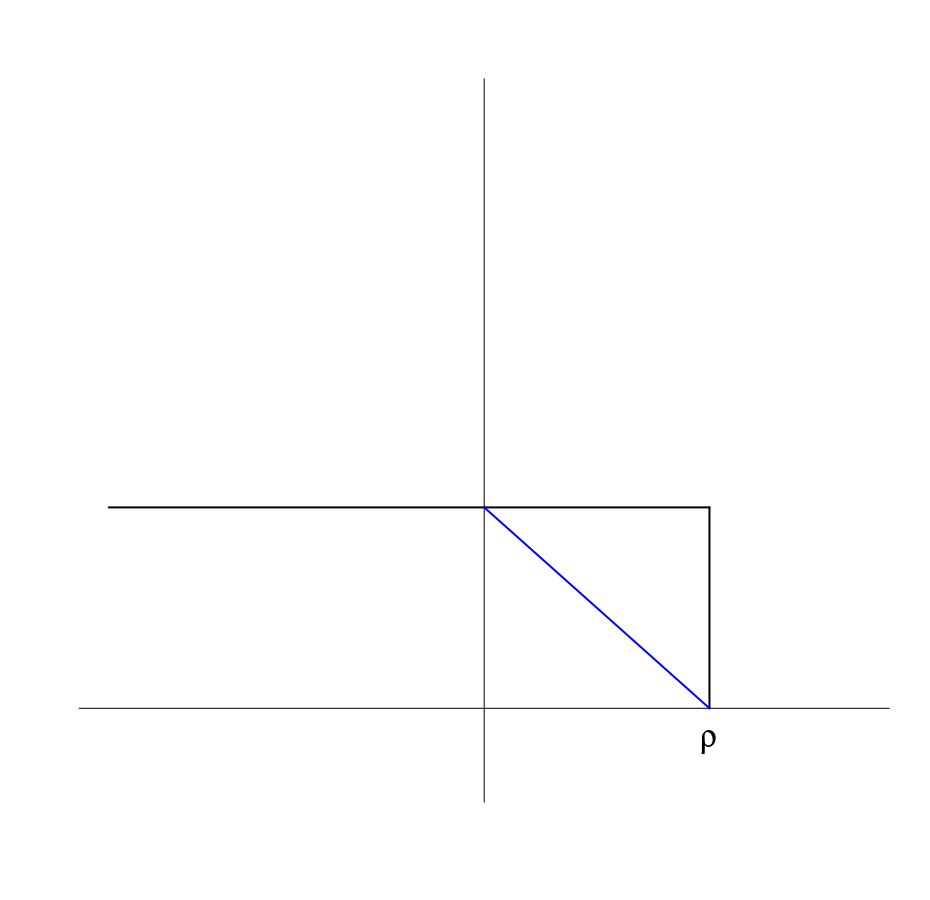At first, I try to use xaxt option to remove the axis, but the box still remains, just same as the one question in the StackOverflow, and I found a possible solution, directly use

plot.new()


All is well before I tried to add the text $\rho$, if I use

text(0.8, 0, expression(rho), cex = 2)


it is OK, but it is exactly on the axis, not proper, but when I tried smaller $y$-coordinate, such as -0.1, the text cannot appear, which seems out of the figure. I have tried par() parameters, such as mar, but does not work.

Then I have no idea, and do not know how to google it. And even though I want to post an issue in the StackOverflow. But a random reference give me ideas, in which the example saves me,

> plot.new()
> plot.window(xlim=c(0,1), ylim=c(5,10))
> abline(a=6, b=3)
> axis(1)
> axis(2)
> title(main="The Overall Title")
> title(xlab="An x-axis label")
> title(ylab="A y-axis label")
> box()


Then I realized that I should add

plot.window(xlim = c(0, 1), ylim = c(-0.1, 0.9))


### smooth curve¶

x <- 1:10
y <- c(2,4,6,8,7,12,14,16,18,20)
lo <- loess(y~x)
plot(x,y)
lines(predict(lo), col='red', lwd=2)


### margin¶

• mar 调节单张图的 margin
• oma 调节整张图外部的 margin

### custom panels in pairs¶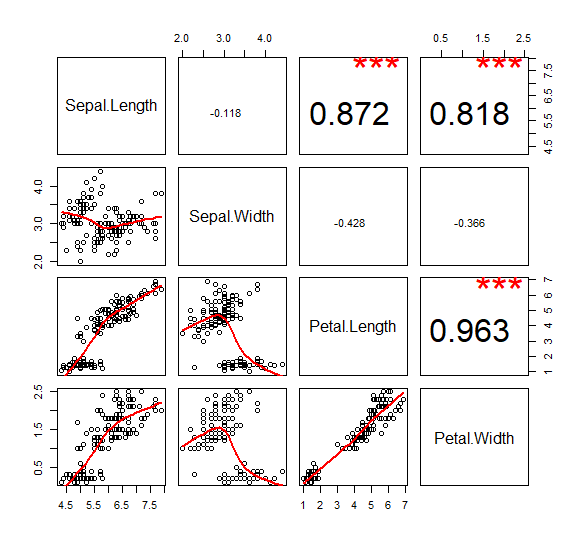my.lower <- function(x,y,...){
points(x, y)
lines(lowess(x, y), col = "red", lwd=2)
}

my.upper <- function(x, y, ...){
cor.val = round(cor(x,y), digits = 3)
if (abs(cor.val) > 0.5){
text(mean(x), mean(y), cor.val, cex = 3)
text(sort(x)[length(x)*0.8], max(y), '***', cex = 4, col = "red")
} else
{
text(mean(x), mean(y), cor.val, cex = 1)
}
}

pairs(iris[1:4], lower.panel =my.lower, upper.panel = my.upper)


### remove outliers from the boxplot¶

How to remove outliers from a dataset

### 在grid排列图¶

Arranging plots in a grid

## lattice¶

The package can easily generate trellis graphs. A trellis graph displays the distribution of a variable or the relationship between variables, separately for each level of one or more other variables.

A thorough tutorial refers to Reproduce Figures with Lattice – ESL CN

## ggplot¶

### histogram¶

fill (not color) & factor (not numeric) in histogram

df = data.frame(a = c(rnorm(100), rnorm(100) +1), g = rep(1:2, each=100))
ggplot(df, aes(a, colour = g)) + geom_histogram()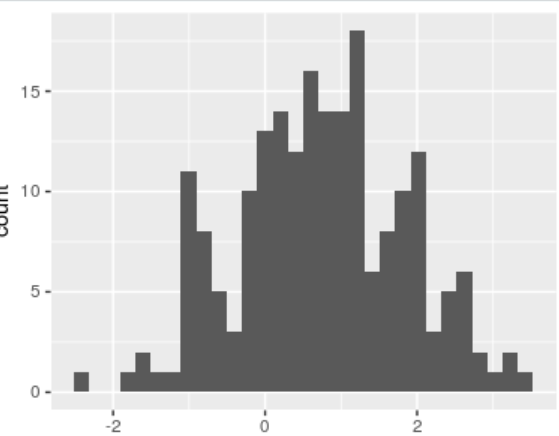ggplot(df, aes(a, col = factor(g) )) + geom_histogram()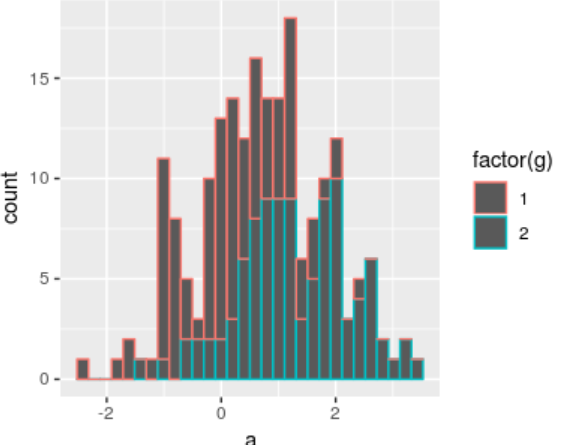ggplot(df, aes(a, fill = factor(g) )) + geom_histogram()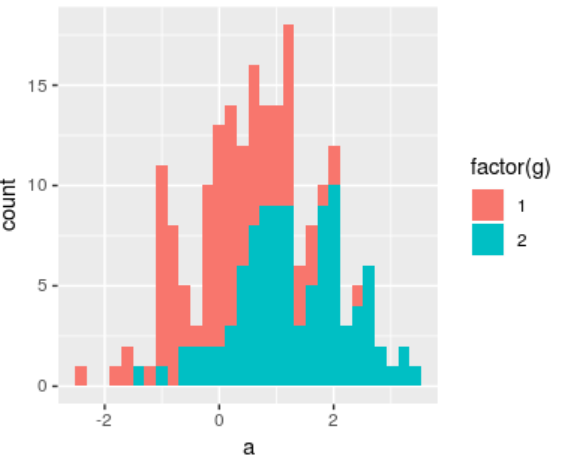alpha not work in single histogram

ggplot(df, aes(a, fill= factor(g)), alpha=0.2) + geom_histogram()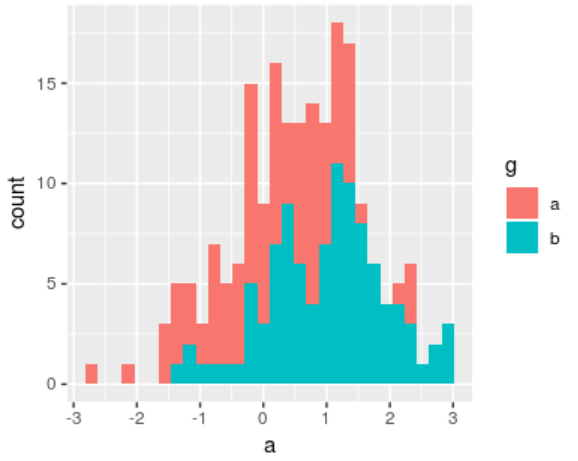ggplot(df, aes(a)) + geom_histogram(data = subset(df, g == 1), aes(fill = factor(g)), alpha = 0.5) +
geom_histogram(data = subset(df, g == 2), aes(fill = factor(g)), alpha = 0.5)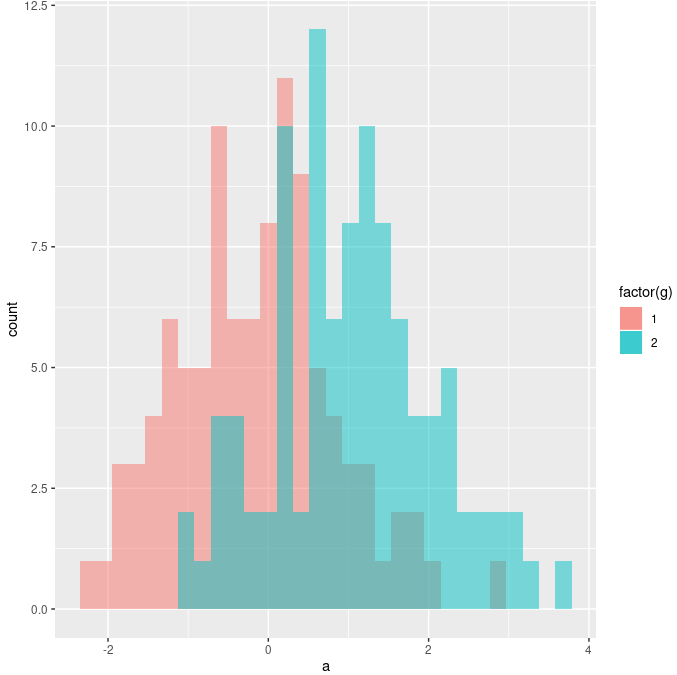Note that aes(fill = ) is important, otherwise no legend. See also:,,scale_fill_manual: do not specify color in aes

If using scale_fill_manual, do not explicitly specify color in aes,

# not recommended
ggplot(df, aes(x)) + geom_histogram(data = subset(df, g=="1"), aes(fill="red"), alpha=0.5) +
geom_histogram(data = subset(df, g=="2"), aes(fill="blue"), alpha=0.5) +
scale_fill_manual(values=c("blue", "red"), labels=c("1", "2"))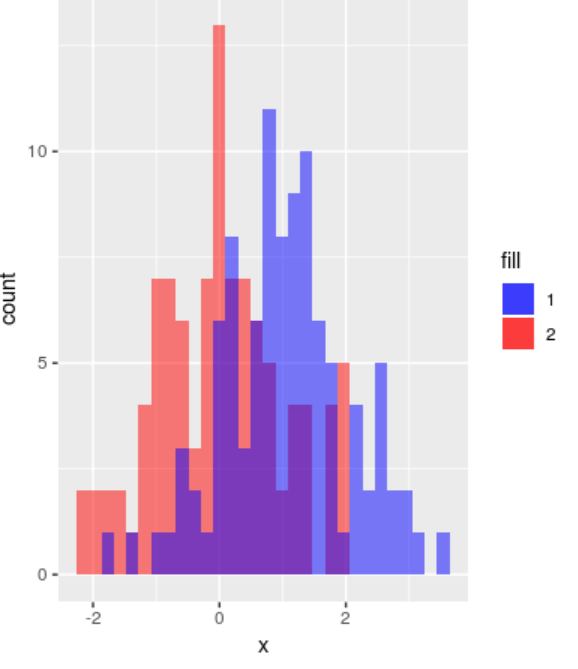Instead, just write the corresponding tuples in values and labels and use fill=g

ggplot(df, aes(x)) + geom_histogram(data = subset(df, g=="1"), aes(fill=g), alpha=0.5) +
geom_histogram(data = subset(df, g=="2"), aes(fill=g), alpha=0.5) +
scale_fill_manual(values=c("blue", "red"), labels=c("1", "2"))


square figure: coord_equal with xlim/ylim

Only coord_equal is not enough.

> df = data.frame(x = runif(10), y = 0.5*runif(10))
> ggplot(df, aes(x, y)) + geom_point() + geom_abline(slope=1) + coord_equal() + xlim(c(0, 1)) + ylim(c(0, 1))

hollow symbol: fill = NAbut note that the default shape=19 (solid disc) does not support fill, so use shape=21 instead.

### multiple density plots¶

plots <- NULL
for (i in 1:4) {
x = i + rnorm(100)
plots[[i]] <- ggplot(data.frame(x), aes(x)) +
geom_density(alpha = 0.5, show.legend = FALSE)
}
cowplot::plot_grid(plotlist = plots)


Application

See one of my homework written in Rmarkdown, 中心极限定理模拟实验

### density of Weibull¶

Take the Weibull distribution as an example,

f(x) = \begin{cases} \frac k\lambda \left(\frac x\lambda\right)^{k-1}e^{-(x/\lambda)^k} & x\ge 0\\ 0 & x < 0 \end{cases}

where $\lambda > 0$ is the scale parameter, and $k > 0$ is the shape parameter. And

• if $k=1$, it becomes to the exponential distribution
• if $k=2$, it becomes to the Rayleigh distribution.
d <- seq(0, 5, length.out=10000)
y <- dweibull(d, shape=5, scale=1, log = FALSE)
df <- data.frame(x=d,y)
ggplot(df, aes(x=d, y=y)) +
geom_line(col = "orange") +
ggtitle("Density of Weibull distribution")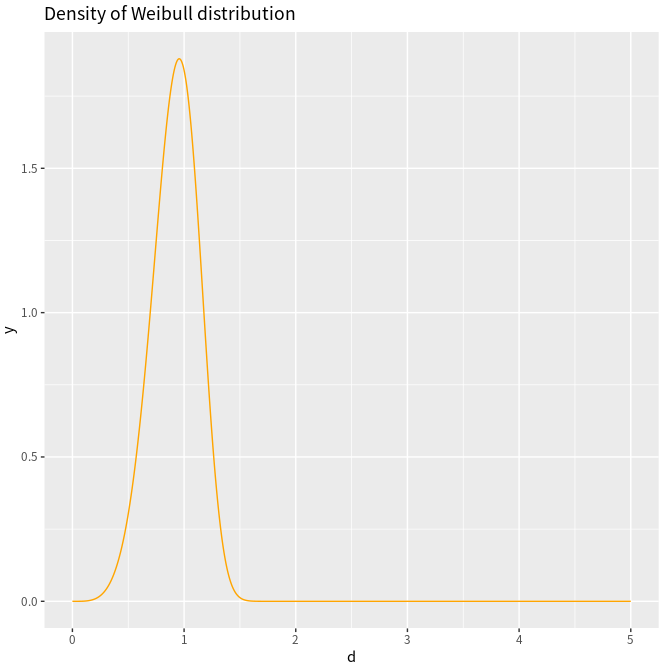h = rweibull(10000, shape=5, scale=1)
ggplot(NULL, aes(x=h)) +
geom_histogram(binwidth=0.01, fill="white", col="red") +
ggtitle("Histogram of Weibull distribution")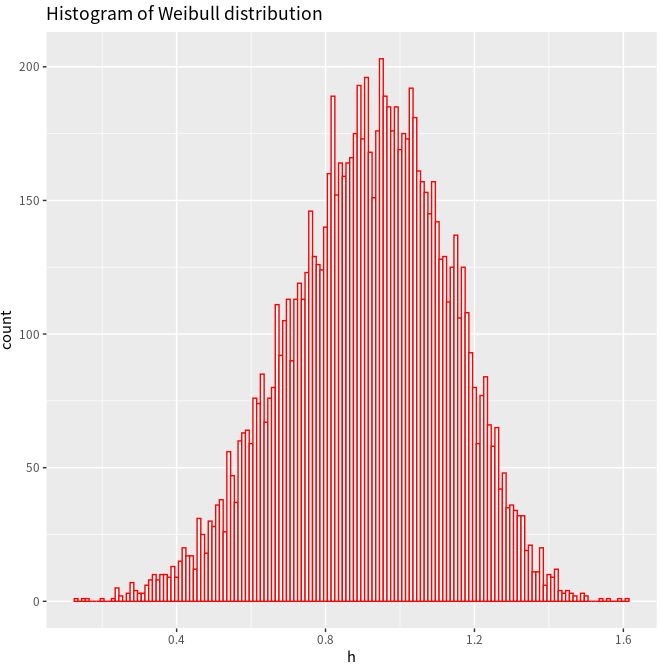ggplot(NULL, aes(x=h)) + geom_density(col = "green")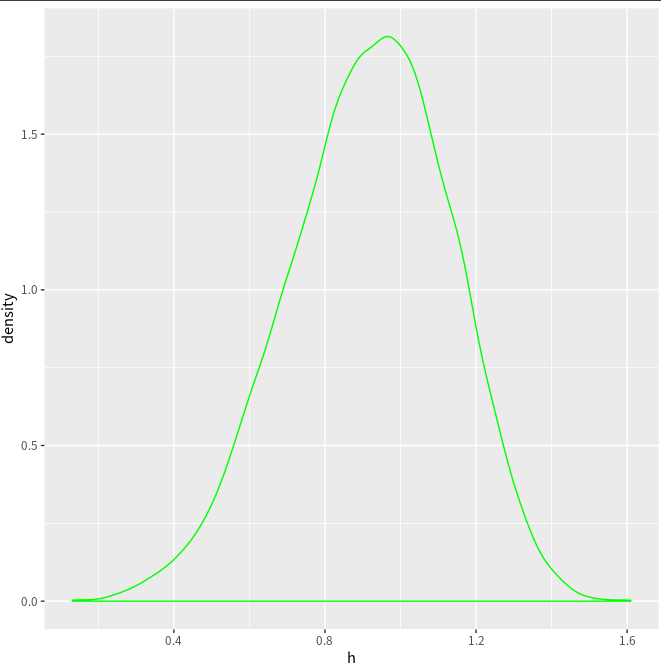ggplot(NULL, aes(x=h)) + geom_line(stat = "density", col = "red")


A minor difference is that here is a horizontal line in the above estimated density.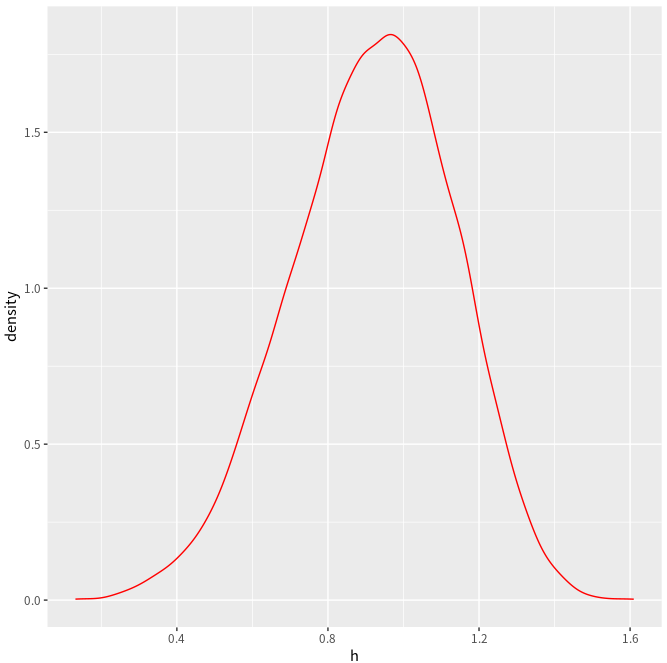Also refer to Plotting distributions (ggplot2)

### legend setup¶

#### 默认情形¶

library(ggplot2)
bp <- ggplot(data=PlantGrowth, aes(x=group, y=weight, fill=group)) + geom_boxplot()
bp


#### 自定义图例的顺序¶

# Remove legend for a particular aesthetic (fill)
bp + guides(fill=FALSE)

# It can also be done when specifying the scale
bp + scale_fill_discrete(guide=FALSE)

# This removes all legends
bp + theme(legend.position="none")


bp + scale_fill_discrete(breaks=c("trt1","ctrl","trt2"))


#### 颠倒图例的顺序¶

# These two methods are equivalent:
bp + guides(fill = guide_legend(reverse=TRUE))
bp + scale_fill_discrete(guide = guide_legend(reverse=TRUE))

# You can also modify the scale directly:
bp + scale_fill_discrete(breaks = rev(levels(PlantGrowth\$group)))


#### 隐藏图例标题¶

# Remove title for fill legend
bp + guides(fill=guide_legend(title=NULL))

# Remove title for all legends
bp + theme(legend.title=element_blank())


#### 自定义图例的标题及名称¶




#### 图例的整体形状¶

# Title appearance
bp + theme(legend.title = element_text(colour="blue", size=16, face="bold"))

# Label appearance
bp + theme(legend.text = element_text(colour="blue", size = 16, face = "bold"))


bp + theme(legend.background = element_rect())
bp + theme(legend.background = element_rect(fill="gray90", size=.5, linetype="dotted"))


bp + theme(legend.position="top")

# Position legend in graph, where x,y is 0,0 (bottom left) to 1,1 (top right)
bp + theme(legend.position=c(.5, .5))

# Set the "anchoring point" of the legend (bottom-left is 0,0; top-right is 1,1)
# Put bottom-left corner of legend box in bottom-left corner of graph
bp + theme(legend.justification=c(0,0), legend.position=c(0,0))

# Put bottom-right corner of legend box in bottom-right corner of graph
bp + theme(legend.justification=c(1,0), legend.position=c(1,0))


#### 隐藏图例的slashes¶

# No outline
ggplot(data=PlantGrowth, aes(x=group, fill=group)) +
geom_bar()

# Add outline, but slashes appear in legend
ggplot(data=PlantGrowth, aes(x=group, fill=group)) +
geom_bar(colour="black")

# A hack to hide the slashes: first graph the bars with no outline and add the legend,
# then graph the bars again with outline, but with a blank legend.
ggplot(data=PlantGrowth, aes(x=group, fill=group)) +
geom_bar() +
geom_bar(colour="black", show.legend=FALSE)


### 多张图片¶

par(mfrow=c(1,2))不起作用，要用到 gridExtra 包，如

library(gridExtra)
plot1 <- qplot(1)
plot2 <- qplot(1)
grid.arrange(plot1, plot2, ncol=2)


### scale_fill_manual 和 scale_color_manual¶

scale_fill_manual(values = c("red", "blue"))


### save¶

NOT png()...dev.off(), use

ggsave("sth.eps",device="eps", width=9)


### aes_string vs aes¶

Last update: May 5, 2023
Created: April 24, 2018